Download Presentation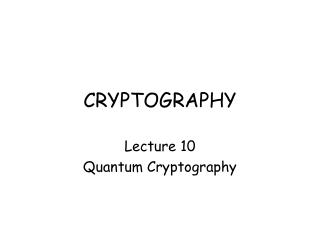CRYPTOGRAPHY

# CRYPTOGRAPHY - PowerPoint PPT Presentation

CRYPTOGRAPHY. Lecture 10 Quantum Cryptography. Quantum Computers for Cryptanalysis. Nobody understands quantum theory. - Richard Feynman, Nobel prize-winning physicistI am the owner, or an agent authorized to act on behalf of the owner, of the copyrighted work described.
Download Presentation## CRYPTOGRAPHY

An Image/Link below is provided (as is) to download presentation

Download Policy: Content on the Website is provided to you AS IS for your information and personal use and may not be sold / licensed / shared on other websites without getting consent from its author.While downloading, if for some reason you are not able to download a presentation, the publisher may have deleted the file from their server.

- - - - - - - - - - - - - - - - - - - - - - - - - - E N D - - - - - - - - - - - - - - - - - - - - - - - - - -
Presentation Transcript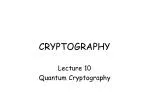### CRYPTOGRAPHY

Lecture 10

Quantum Cryptography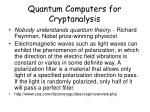Quantum Computers for Cryptanalysis
• Nobody understands quantum theory. - Richard Feynman, Nobel prize-winning physicist
• Electromagnetic waves such as light waves can exhibit the phenomenon of polarization, in which the direction of the electric field vibrations is constant or varies in some definite way. A polarization filter is a material that allows only light of a specified polarization direction to pass. If the light is randomly polarized, only half of it will pass a perfect filter.
• http://www.csa.com/discoveryguides/crypt/overview.php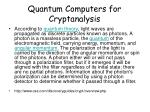Quantum Computers for Cryptanalysis
• According to quantum theory, light waves are propagated as discrete particles known as photons. A photon is a massless particle, the quantum of the electromagnetic field, carrying energy, momentum, and angular momentum. The polarization of the light is carried by the direction of the angular momentum or spin of the photons. A photon either will or will not pass through a polarization filter, but if it emerges it will be aligned with the filter regardless of its inital state; there are no partial photons. Information about the photon's polarization can be determined by using a photon detector to determine whether it passed through a filter.
• http://www.csa.com/discoveryguides/crypt/overview.php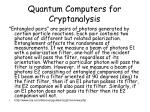Quantum Computers for Cryptanalysis

"Entangled pairs" are pairs of photons generated by certain particle reactions. Each pair contains two photons of different but related polarization. Entanglement affects the randomness of measurements. If we measure a beam of photons E1 with a polarization filter, one-half of the incident photons will pass the filter, regardless of its orientation. Whether a particular photon will pass the filter is random. However, if we measure a beam of photons E2 consisting of entangled companions of the E1 beam with a filter oriented at 90 degrees (deg) to the first filter, then if an E1 photon passes its filter, its E2 companion will also pass its filter. Similarly, if an E1 photon does not pass its filter then its E2 companion will not.

• http://www.csa.com/discoveryguides/crypt/overview.php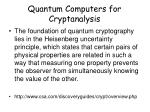Quantum Computers for Cryptanalysis
• The foundation of quantum cryptography lies in the Heisenberg uncertainty principle, which states that certain pairs of physical properties are related in such a way that measuring one property prevents the observer from simultaneously knowing the value of the other.
• http://www.csa.com/discoveryguides/crypt/overview.php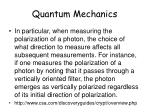Quantum Mechanics
• In particular, when measuring the polarization of a photon, the choice of what direction to measure affects all subsequent measurements. For instance, if one measures the polarization of a photon by noting that it passes through a vertically oriented filter, the photon emerges as vertically polarized regardless of its initial direction of polarization.
• http://www.csa.com/discoveryguides/crypt/overview.php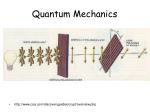Quantum Mechanics
• http://www.csa.com/discoveryguides/crypt/overview.php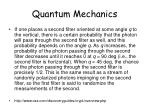Quantum Mechanics
• If one places a second filter oriented at some angle q to the vertical, there is a certain probability that the photon will pass through the second filter as well, and this probability depends on the angle q. As q increases, the probability of the photon passing through the second filter decreases until it reaches 0 at q = 90 deg (i.e., the second filter is horizontal). When q = 45 deg, the chance of the photon passing through the second filter is precisely 1/2. This is the same result as a stream of randomly polarized photons impinging on the second filter, so the first filter is said to randomize the measurements of the second.
• http://www.csa.com/discoveryguides/crypt/overview.php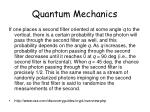Quantum Mechanics

If one places a second filter oriented at some angle q to the vertical, there is a certain probability that the photon will pass through the second filter as well, and this probability depends on the angle q. As q increases, the probability of the photon passing through the second filter decreases until it reaches 0 at q = 90 deg (i.e., the second filter is horizontal). When q = 45 deg, the chance of the photon passing through the second filter is precisely 1/2. This is the same result as a stream of randomly polarized photons impinging on the second filter, so the first filter is said to randomize the measurements of the second.

• http://www.csa.com/discoveryguides/crypt/overview.php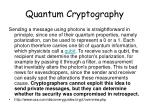Quantum Cryptography

Sending a message using photons is straightforward in principle, since one of their quantum properties, namely polarization, can be used to represent a 0 or a 1. Each photon therefore carries one bit of quantum information, which physicists call a qubit. To receive such a qubit, the recipient must determine the photon's polarization, for example by passing it through a filter, a measurement that inevitably alters the photon's properties. This is bad news for eavesdroppers, since the sender and receiver can easily spot the alterations these measurements cause. Cryptographers cannot exploit this idea to send private messages, but they can determine whether its security was compromised in retrospect.

• http://www.csa.com/discoveryguides/crypt/overview.phpQuantum Cryptography

The genius of quantum cryptography is that it solves the problem of key distribution. A user can suggest a key by sending a series of photons with random polarizations. This sequence can then be used to generate a sequence of numbers. The process is known as quantum key distribution. If the key is intercepted by an eavesdropper, this can be detected and it is of no consequence, since it is only a set of random bits and can be discarded. The sender can then transmit another key. Once a key has been securely received, it can be used to encrypt a message that can be transmitted by conventional means: telephone, e-mail, or regular postal mail

• http://www.csa.com/discoveryguides/crypt/overview.phpQuantum Key Cryptography
• Quantum cryptography is an effort to allow two users of a common communication channel to create a body of shared and secret information. This information, which generally takes the form of a random string of bits, can then be used as a conventional secret key for secure communication. It is useful to assume that the communicating parties initially share a small amount of secret information, which is used up and then renewed in the exchange process, but even without this assumption exchanges are possible.

From http://www.cs.dartmouth.edu/~jford/crypto.htmlQuantum Key Cryptography
• The advantage of quantum cryptography over traditional key exchange methods is that the exchange of information can be shown to be secure in a very strong sense, without making assumptions about the intractability of certain mathematical problems. Even when assuming hypothetical eavesdroppers with unlimited computing power, the laws of physics guarantee (probabilistically) that the secret key exchange will be secure, given a few other assumptions.

From http://www.cs.dartmouth.edu/~jford/crypto.htmlQuantum Key Cryptography
• The roots of quantum cryptography are in a proposal by Stephen Weisner called "Conjugate Coding" from the early 1970s. It was eventually published in 1983 in Sigact News, and by that time Bennett and Brassard, who were familiar with Weisner's ideas, were ready to publish ideas of their own. They produced "BB84," the first quantum cryptography protocol, in 1984, but it was not until 1991 that the first experimental prototype based on this protocol was made operable (over a distance of 32 centimeters).

From http://www.cs.dartmouth.edu/~jford/crypto.htmlQuantum Key Cryptography
• Bennett et al.  (see [Henle WWW] for an online demonstration). It uses polarization of photons as its units of information. Polarization can be measured using three different bases, which are conjugates: rectilinear (horizontal or vertical), circular (left-circular or right-circular), and diagonal (45 or 135 degrees). Only the rectilinear and circular bases are used in the protocol, but the diagonal basis is slightly useful for eavesdropping.

From http://www.cs.dartmouth.edu/~jford/crypto.htmlQuantum Key Cryptography
• The light source, often a light-emitting diode (LED) or laser, is filtered to produce a polarized beam in short bursts with a very low intensity. The polarization in each burst is then modulated randomly to one of four states (horizontal, vertical, left-circular, or right-circular) by the sender, Alice.
• The receiver, Bob, measures photon polarizations in a random sequence of bases (rectilinear or circular).
• Bob tells the sender publicly what sequence of bases were used.
• Alice tells the receiver publicly which bases were correctly chosen.
• Alice and Bob discard all observations not from these correctly-chosen bases.
• The observations are interpreted using a binary scheme: left-circular or horizontal is 0, and right-circular or vertical is 1.

From http://www.cs.dartmouth.edu/~jford/crypto.htmlQuantum Key Cryptography
• This protocol is complicated by the presence of noise, which may occur randomly or may be introduced by eavesdropping. When noise exists, polarizations observed by the receiver may not correspond to those emitted by the sender. In order to deal with this possibility, Alice and Bob must ensure that they possess the same string of bits, removing any discrepancies. This is generally done using a binary search with parity checks to isolate differences; by discarding the last bit with each check, the public discussion of the parity is rendered harmless.

From http://www.cs.dartmouth.edu/~jford/crypto.htmlQuantum Key Cryptography
• The sender, Alice, and the receiver, Bob, agree on a random permutation of bit positions in their strings (to randomize the location of errors).
• The strings are partitioned into blocks of size k (k ideally chosen so that the probability of multiple errors per block is small).
• For each block, Alice and Bob compute and publicly announce parities. The last bit of each block is then discarded.
• For each block for which their calculated parities are different, Alice and Bob use a binary search with log(k) iterations to locate and correct the error in the block.
• To account for multiple errors that might remain undetected, steps 1-4 are repeated with increasing block sizes in an attempt to eliminate these errors.
• To determine whether additional errors remain, Alice and Bob repeat a randomized check:
• Alice and Bob agree publicly on a random assortment of half the bit positions in their bit strings.
• Alice and Bob publicly compare parities (and discard a bit). If the strings differ, the parities will disagree with probability 1/2.
• If there is disagreement, Alice and Bob use a binary search to find and eliminate it, as above.
• If there is no disagreement after l iterations, Alice and Bob conclude their strings agree with low probability of error (2^-l).

From http://www.cs.dartmouth.edu/~jford/crypto.htmlQuantum Key Cryptography
• The most straightforward application of quantum cryptography is in distribution of secret keys. The amount of information that can be transmitted is not very large, but it is provably very secure. By taking advantage of existing secret-key cryptographic algorithms, this initial transfer can be leveraged to achieve a secure transmission of large amounts of data at much higher speeds. Quantum cryptography is thus an excellent replacement for the Diffie-Hellman key exchange algorithm mentioned above.Quantum Key Cryptography
• The elements of quantum information exchange are observations of quantum states; typically photons are put into a particular state by the sender and then observed by the recipient. Because of the Uncertainty Principle, certain quantum information occurs as conjugates that cannot be measured simultaneously. Depending on how the observation is carried out, different aspects of the system can be measured -- for example, polarizations of photons can be expressed in any of three different bases: rectilinear, circular, and diagonal -- but observing in one basis randomizes the conjugates. Thus, if the receiver and sender do not agree on what basis of a quantum system they are using as bases, the receiver may inadvertently destroy the sender's information without gaining anything useful.Quantum Key Cryptography
• This, then, is the overall approach to quantum transmission of information: the sender encodes it in quantum states, the receiver observes these states, and then by public discussion of the observations the sender and receiver agree on a body of information they share (with arbitrarily high probability). Their discussion must deal with errors, which may be introduced by random noise or by eavesdroppers, but must be general, so as not to compromise the information. This may be accomplished by discussing parities of bits (*) rather than individual bits; by afterwards discarding an agreed-upon bit, such as the last one, a parity can then be made useless to eavesdroppers.Quantum Key Cryptography
• Once the secret bit string is agreed to, the technique of privacy amplification can be used to reduce an outsider's potential knowledge of it to an arbitrarily low level. If an eavesdropper knows l "deterministic bits" (e.g., bits of the string, or parity bits) of the length n string x, then a randomly and publicly chosen hash function, h, can be used to map the string x onto a new string h(x) of length n - l - s for any selected positive s. It can then be shown that the eavesdropper's expected knowledge of h(x) is less than 2^-s/ln2 bits.Quantum Key Cryptography
• Quantum cryptographic techniques provide no protection against the classic bucket brigade attack (also known as the "man-in-the-middle attack"). In this scheme, an eavesdropper, E ("Eve") is assumed to have the capacity to monitor the communications channel and insert and remove messages without inaccuracy or delay. When Alice attempts to establish a secret key with Bob, Eve intercepts and responds to messages in both directions, fooling both Alice and Bob into believing she is the other. Once the keys are established, Eve receives, copies, and resends messages so as to allow Alice and Bob to communicate. Assuming that processing time and accuracy are not difficulties, Eve will be able to retrieve the entire secret key -- and thus the entire plaintext of every message sent between Alice and Bob -- without any detectable signs of eavesdropping.